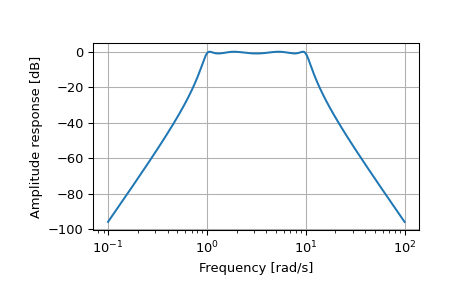# scipy.signal.freqs¶

scipy.signal.freqs(b, a, worN=200, plot=None)[source]

Compute frequency response of analog filter.

Given the M-order numerator b and N-order denominator a of an analog filter, compute its frequency response:

```        b*(jw)**M + b*(jw)**(M-1) + ... + b[M]
H(w) = ----------------------------------------------
a*(jw)**N + a*(jw)**(N-1) + ... + a[N]
```
Parameters
barray_like

Numerator of a linear filter.

aarray_like

Denominator of a linear filter.

worN{None, int, array_like}, optional

If None, then compute at 200 frequencies around the interesting parts of the response curve (determined by pole-zero locations). If a single integer, then compute at that many frequencies. Otherwise, compute the response at the angular frequencies (e.g., rad/s) given in worN.

plotcallable, optional

A callable that takes two arguments. If given, the return parameters w and h are passed to plot. Useful for plotting the frequency response inside `freqs`.

Returns
wndarray

The angular frequencies at which h was computed.

hndarray

The frequency response.

`freqz`

Compute the frequency response of a digital filter.

Notes

Using Matplotlib’s “plot” function as the callable for plot produces unexpected results, this plots the real part of the complex transfer function, not the magnitude. Try `lambda w, h: plot(w, abs(h))`.

Examples

```>>> from scipy.signal import freqs, iirfilter
```
```>>> b, a = iirfilter(4, [1, 10], 1, 60, analog=True, ftype='cheby1')
```
```>>> w, h = freqs(b, a, worN=np.logspace(-1, 2, 1000))
```
```>>> import matplotlib.pyplot as plt
>>> plt.semilogx(w, 20 * np.log10(abs(h)))
>>> plt.xlabel('Frequency')
>>> plt.ylabel('Amplitude response [dB]')
>>> plt.grid()
>>> plt.show()
```